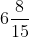# ML Aggarwal Solutions for Class 7 Maths Chapter 2 Fractions and Decimals

ML Aggarwal Solutions for Class 7 Maths Chapter 2 Fractions and Decimals provides answers designed by experienced teachers in a descriptive manner. These solutions help students to analyze the sort of questions that would appear in the final examination. Students who aspire to score high marks are suggested to practice ML Aggarwal Solutions on a regular basis. For more conceptual knowledge, students can make use of ML Aggarwal Solutions for Class 7 Maths Chapter 2 Fractions and Decimals PDF, from the links which are available here.

A number representing a part of a whole is known as a Fraction and the number whose whole number and the fractional part is separated by a decimal point is known as Decimals. This Chapter 2 of ML Aggarwal Solutions for Class 7 is vital as it is continued in further classes also. The solutions help students to understand the concepts in an effective way.

## ML Aggarwal Solutions for Class 7 Maths Chapter 2: Fractions and Decimals Download PDF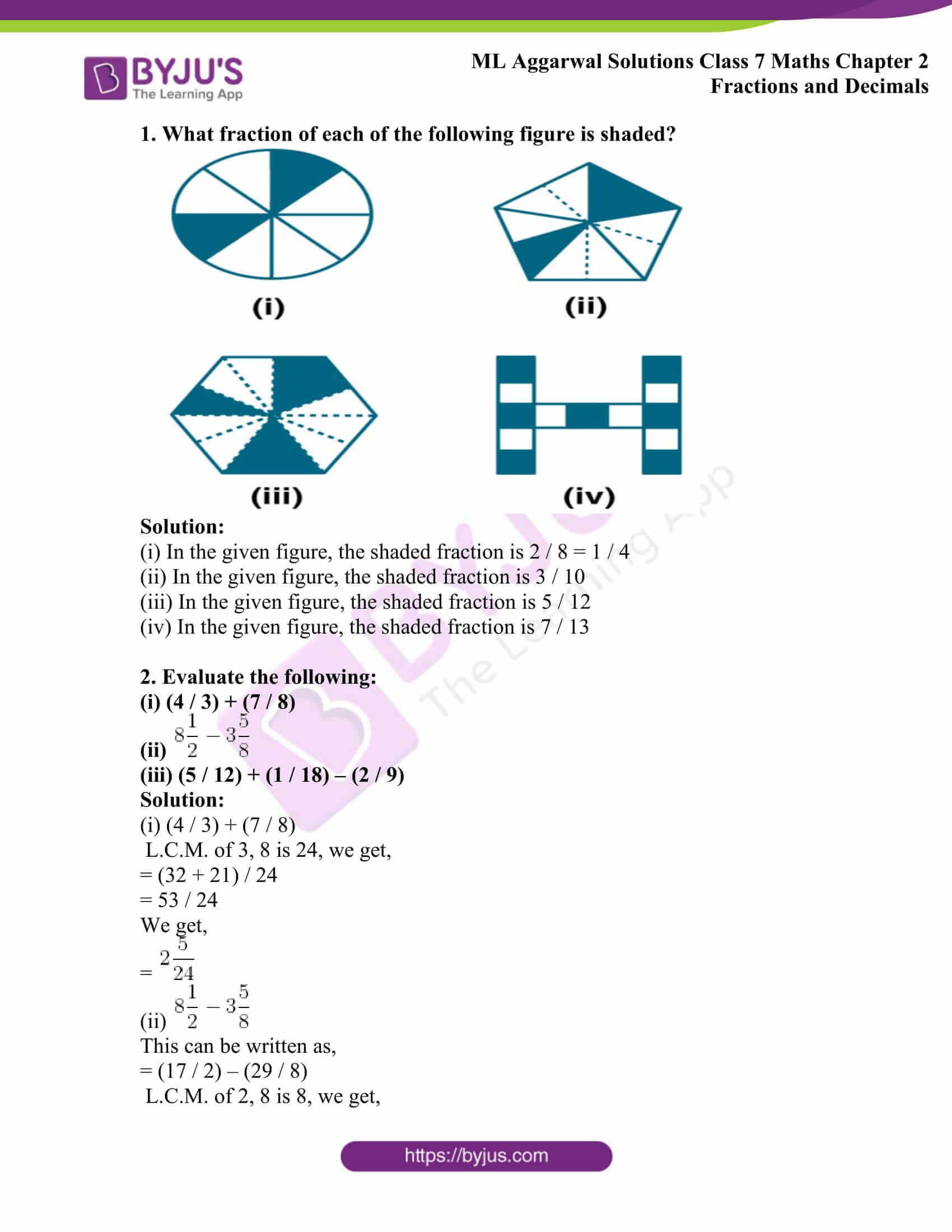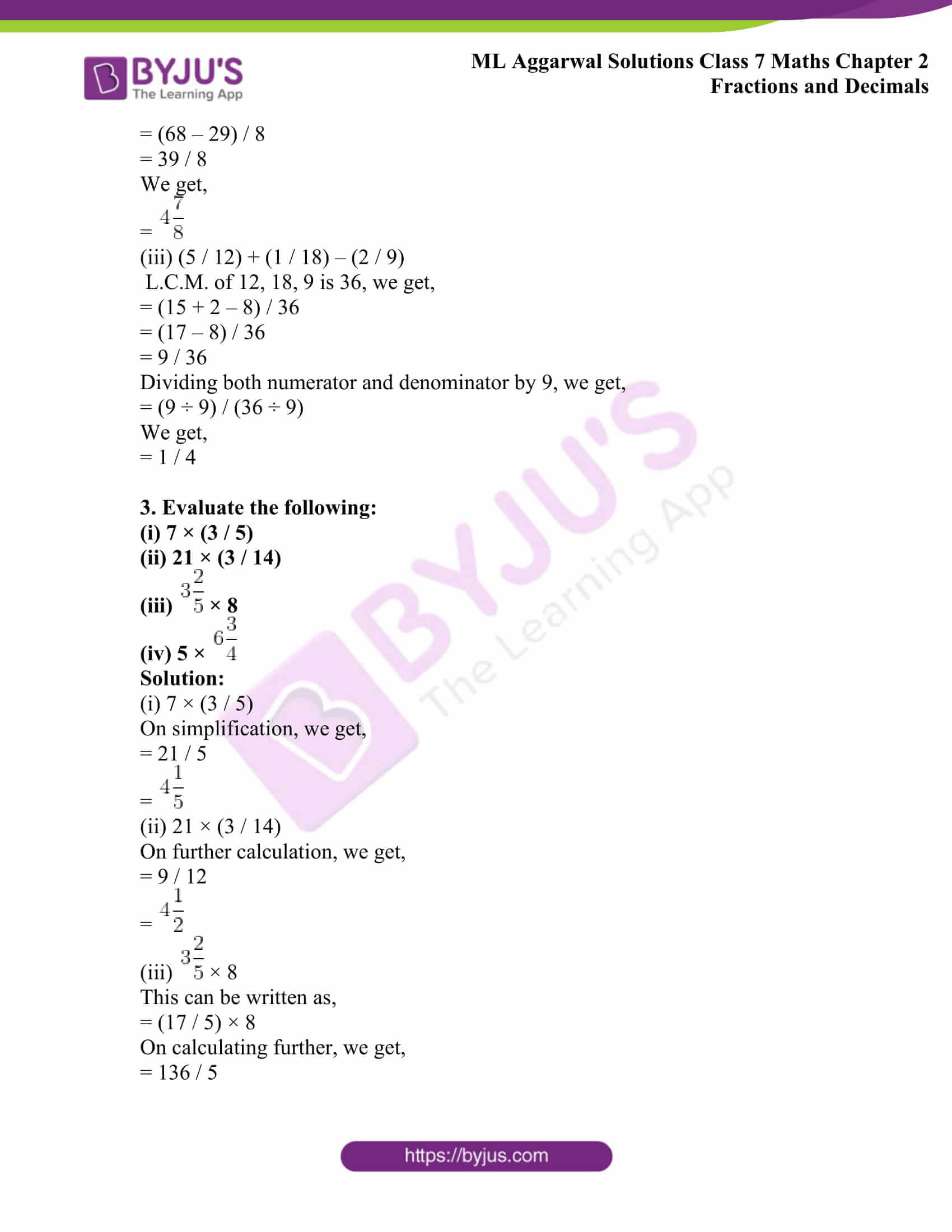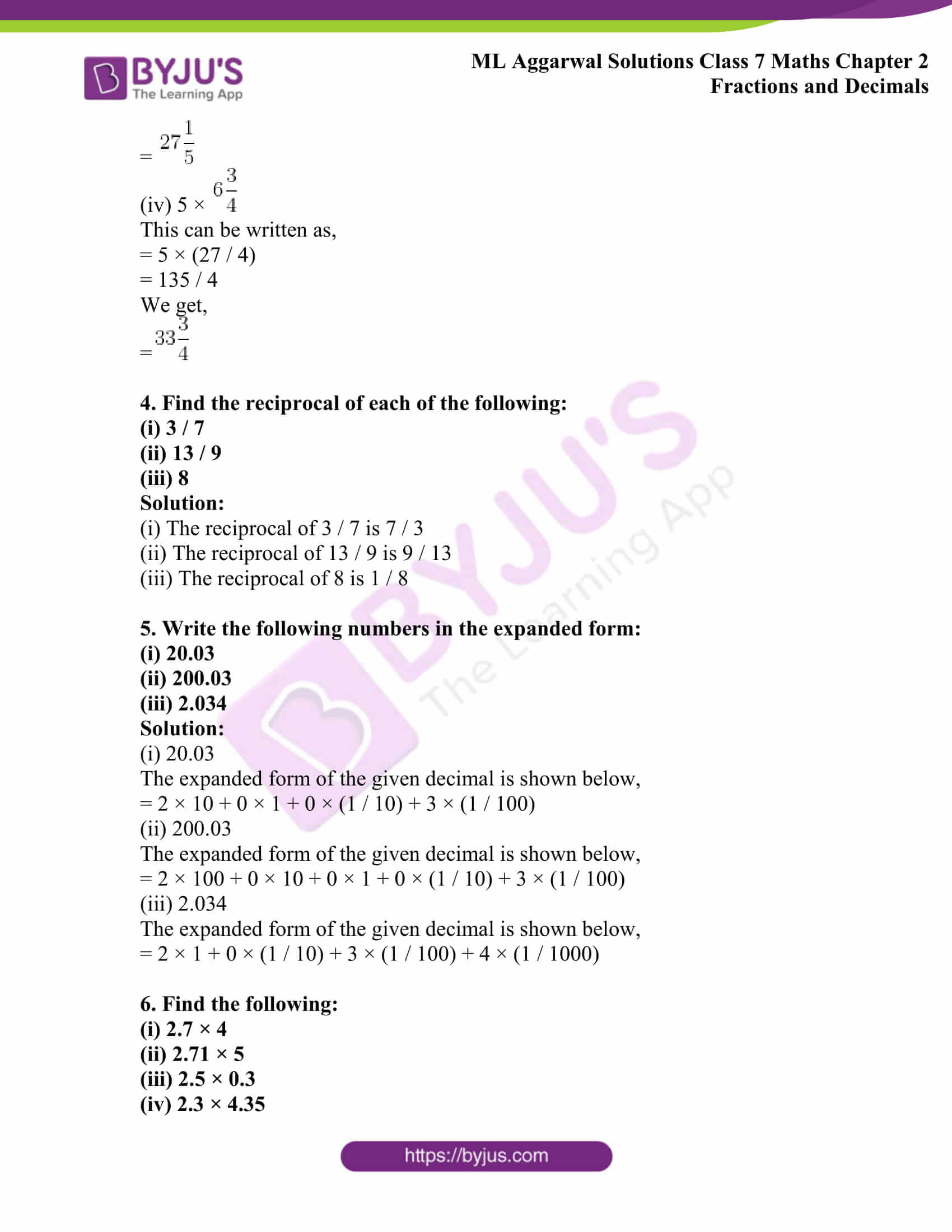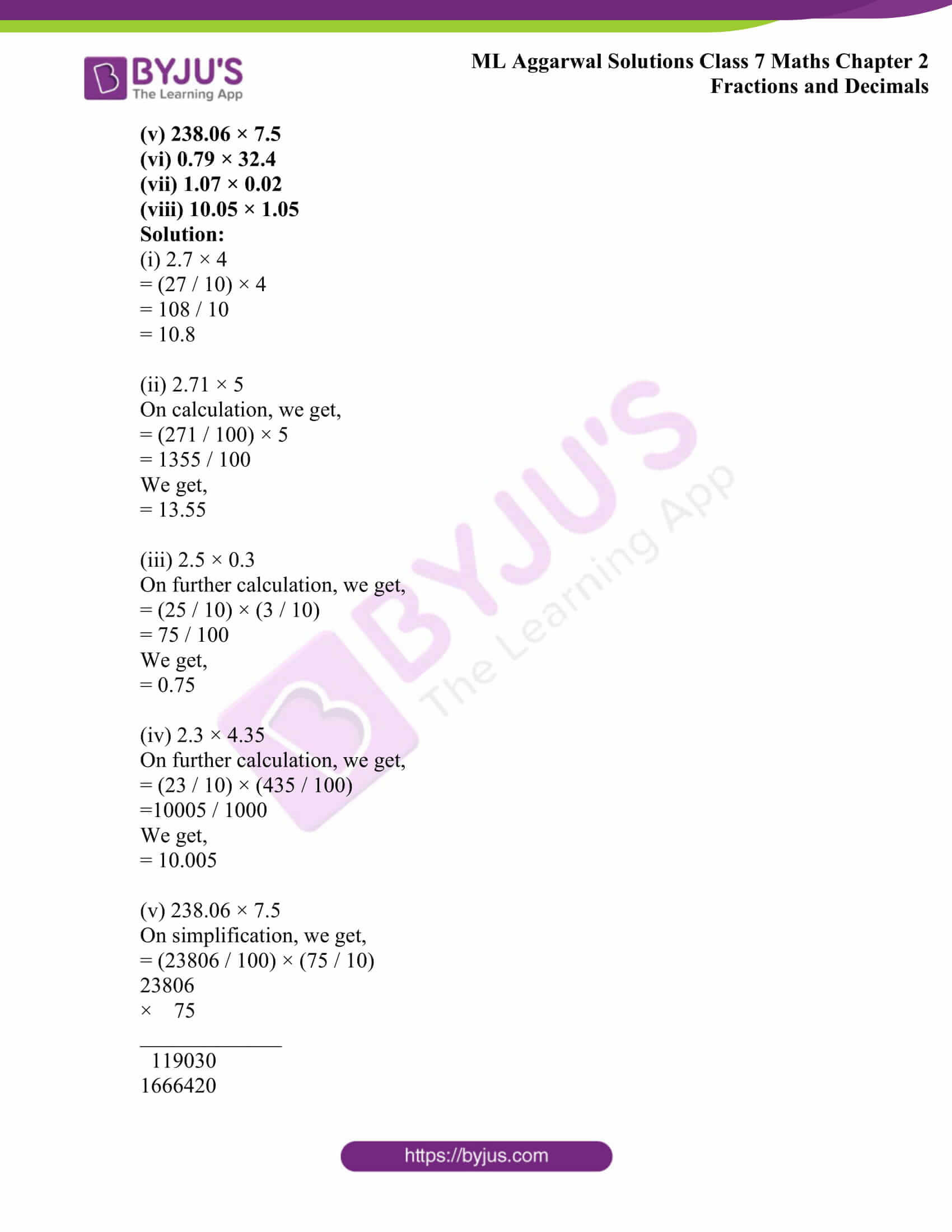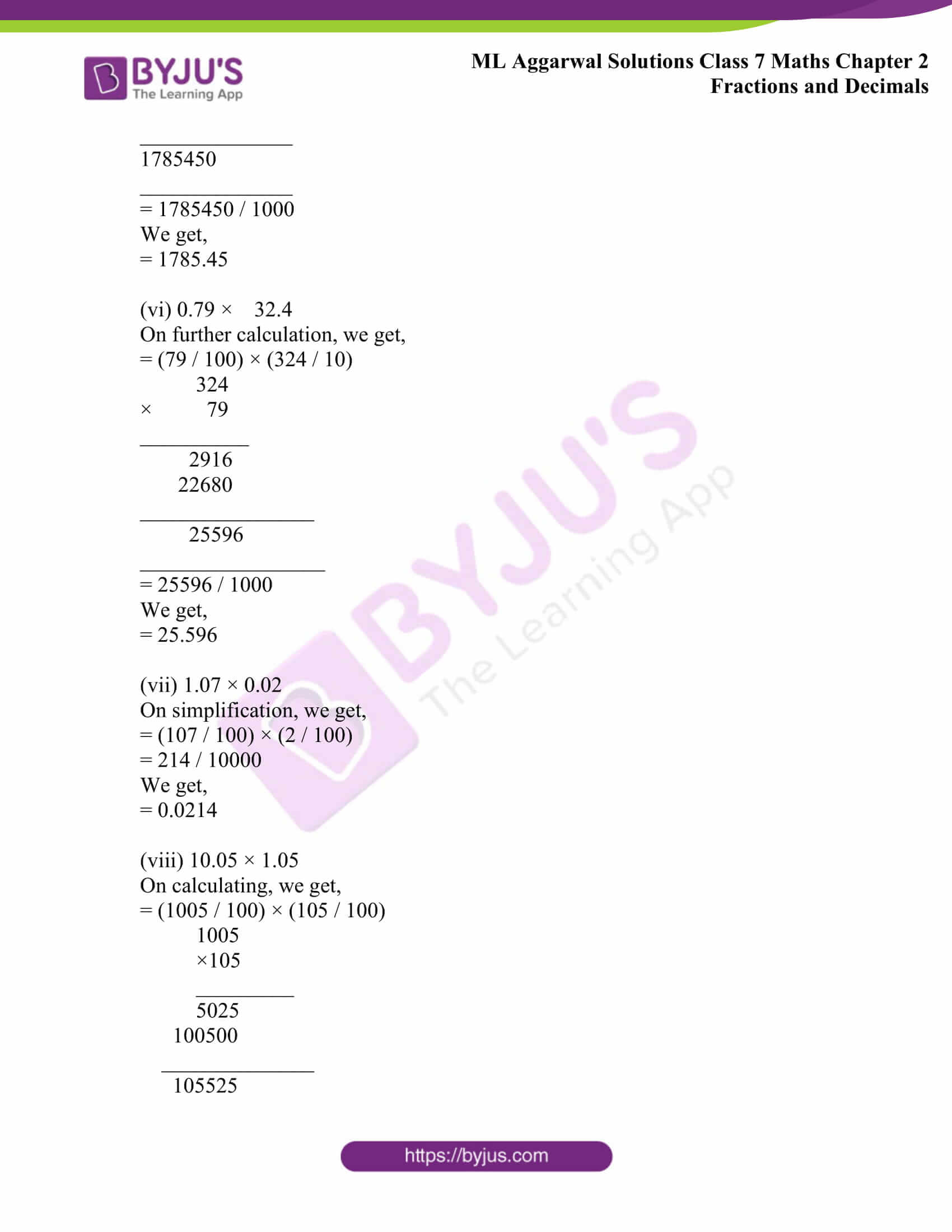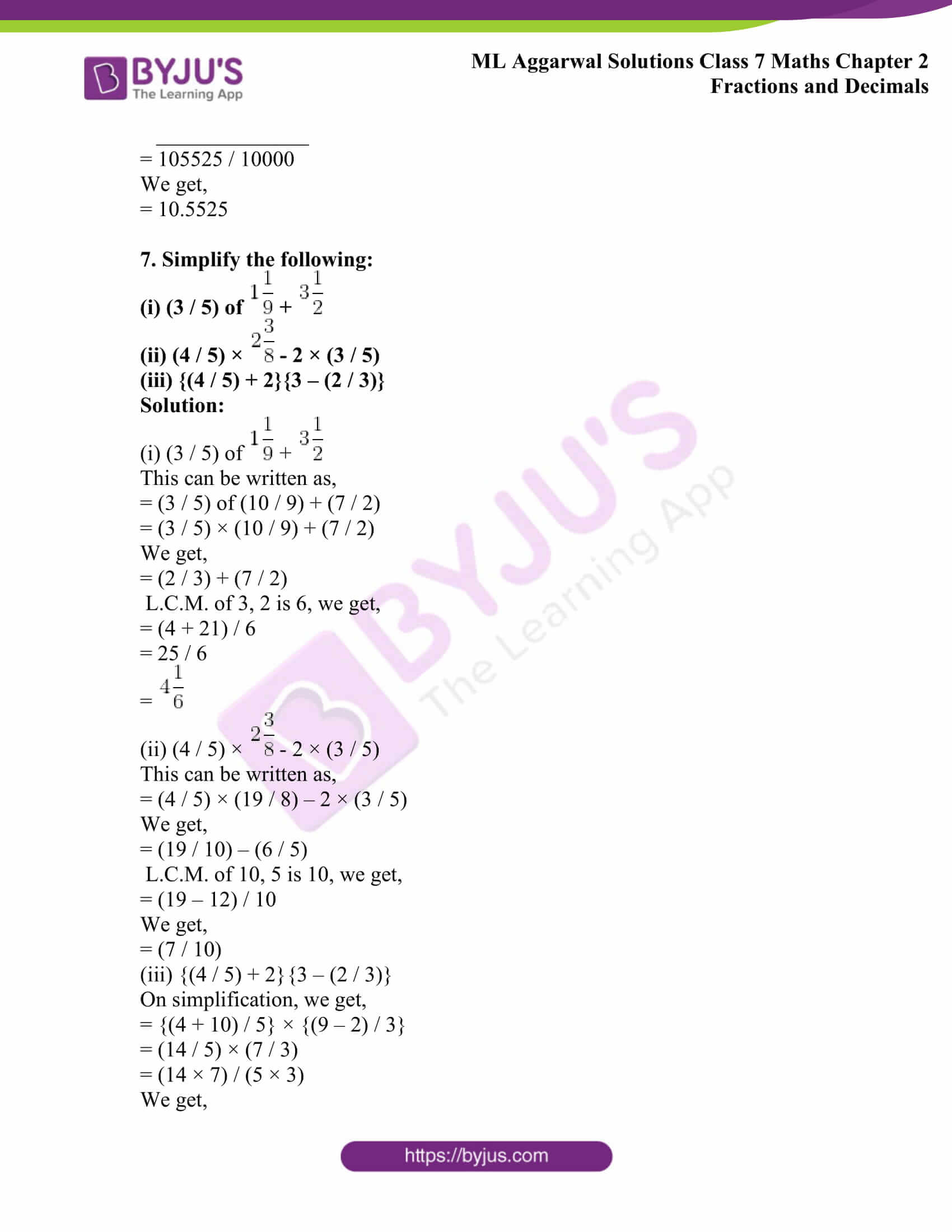## Access ML Aggarwal Solutions for Class 7 Maths Chapter 2: Fractions and Decimals

1. What fraction of each of the following figure is shaded?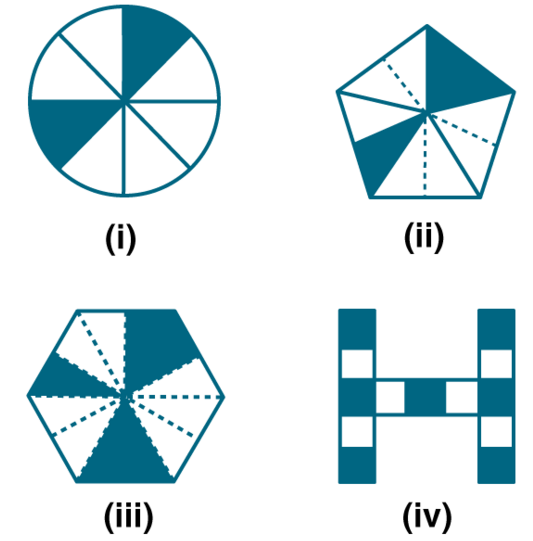Solution:

(i) In the given figure, the shaded fraction is 2 / 8 = 1 / 4

(ii) In the given figure, the shaded fraction is 3 / 10

(iii) In the given figure, the shaded fraction is 5 / 12

(iv) In the given figure, the shaded fraction is 7 / 13

2. Evaluate the following:

(i) (4 / 3) + (7 / 8)

(ii)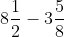(iii) (5 / 12) + (1 / 18) – (2 / 9)

Solution:

(i) (4 / 3) + (7 / 8)

L.C.M. of 3, 8 is 24, we get,

= (32 + 21) / 24

= 53 / 24

We get,

=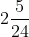(ii)This can be written as,

= (17 / 2) – (29 / 8)

L.C.M. of 2, 8 is 8, we get,

= (68 – 29) / 8

= 39 / 8

We get,

=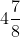(iii) (5 / 12) + (1 / 18) – (2 / 9)

L.C.M. of 12, 18, 9 is 36, we get,

= (15 + 2 – 8) / 36

= (17 – 8) / 36

= 9 / 36

Dividing both numerator and denominator by 9, we get,

= (9 ÷ 9) / (36 ÷ 9)

We get,

= 1 / 4

3. Evaluate the following:

(i) 7 × (3 / 5)

(ii) 21 × (3 / 14)

(iii)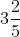× 8

(iv) 5 ×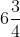Solution:

(i) 7 × (3 / 5)

On simplification, we get,

= 21 / 5

=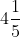(ii) 21 × (3 / 14)

On further calculation, we get,

= 9 / 12

=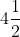(iii)× 8

This can be written as,

= (17 / 5) × 8

On calculating further, we get,

= 136 / 5

=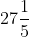(iv) 5 ×This can be written as,

= 5 × (27 / 4)

= 135 / 4

We get,

=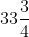4. Find the reciprocal of each of the following:

(i) 3 / 7

(ii) 13 / 9

(iii) 8

Solution:

(i) The reciprocal of 3 / 7 is 7 / 3

(ii) The reciprocal of 13 / 9 is 9 / 13

(iii) The reciprocal of 8 is 1 / 8

5. Write the following numbers in the expanded form:

(i) 20.03

(ii) 200.03

(iii) 2.034

Solution:

(i) 20.03

The expanded form of the given decimal is shown below,

= 2 × 10 + 0 × 1 + 0 × (1 / 10) + 3 × (1 / 100)

(ii) 200.03

The expanded form of the given decimal is shown below,

= 2 × 100 + 0 × 10 + 0 × 1 + 0 × (1 / 10) + 3 × (1 / 100)

(iii) 2.034

The expanded form of the given decimal is shown below,

= 2 × 1 + 0 × (1 / 10) + 3 × (1 / 100) + 4 × (1 / 1000)

6. Find the following:

(i) 2.7 × 4

(ii) 2.71 × 5

(iii) 2.5 × 0.3

(iv) 2.3 × 4.35

(v) 238.06 × 7.5

(vi) 0.79 × 32.4

(vii) 1.07 × 0.02

(viii) 10.05 × 1.05

Solution:

(i) 2.7 × 4

= (27 / 10) × 4

= 108 / 10

= 10.8

(ii) 2.71 × 5

On calculation, we get,

= (271 / 100) × 5

= 1355 / 100

We get,

= 13.55

(iii) 2.5 × 0.3

On further calculation, we get,

= (25 / 10) × (3 / 10)

= 75 / 100

We get,

= 0.75

(iv) 2.3 × 4.35

On further calculation, we get,

= (23 / 10) × (435 / 100)

=10005 / 1000

We get,

= 10.005

(v) 238.06 × 7.5

On simplification, we get,

= (23806 / 100) × (75 / 10)

23806

× 75

_____________

119030

1666420

______________

1785450

______________

= 1785450 / 1000

We get,

= 1785.45

(vi) 0.79 × 32.4

On further calculation, we get,

= (79 / 100) × (324 / 10)

324

× 79

__________

2916

22680

________________

25596

_________________

= 25596 / 1000

We get,

= 25.596

(vii) 1.07 × 0.02

On simplification, we get,

= (107 / 100) × (2 / 100)

= 214 / 10000

We get,

= 0.0214

(viii) 10.05 × 1.05

On calculating, we get,

= (1005 / 100) × (105 / 100)

1005

×105

_________

5025

100500

______________

105525

______________

= 105525 / 10000

We get,

= 10.5525

7. Simplify the following:

(i) (3 / 5) of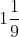+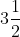(ii) (4 / 5) ×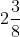– 2 × (3 / 5)

(iii) {(4 / 5) + 2}{3 – (2 / 3)}

Solution:

(i) (3 / 5) of+This can be written as,

= (3 / 5) of (10 / 9) + (7 / 2)

= (3 / 5) × (10 / 9) + (7 / 2)

We get,

= (2 / 3) + (7 / 2)

L.C.M. of 3, 2 is 6, we get,

= (4 + 21) / 6

= 25 / 6

=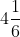(ii) (4 / 5) ×– 2 × (3 / 5)

This can be written as,

= (4 / 5) × (19 / 8) – 2 × (3 / 5)

We get,

= (19 / 10) – (6 / 5)

L.C.M. of 10, 5 is 10, we get,

= (19 – 12) / 10

We get,

= (7 / 10)

(iii) {(4 / 5) + 2}{3 – (2 / 3)}

On simplification, we get,

= {(4 + 10) / 5} × {(9 – 2) / 3}

= (14 / 5) × (7 / 3)

= (14 × 7) / (5 × 3)

We get,

= 98 / 15

=